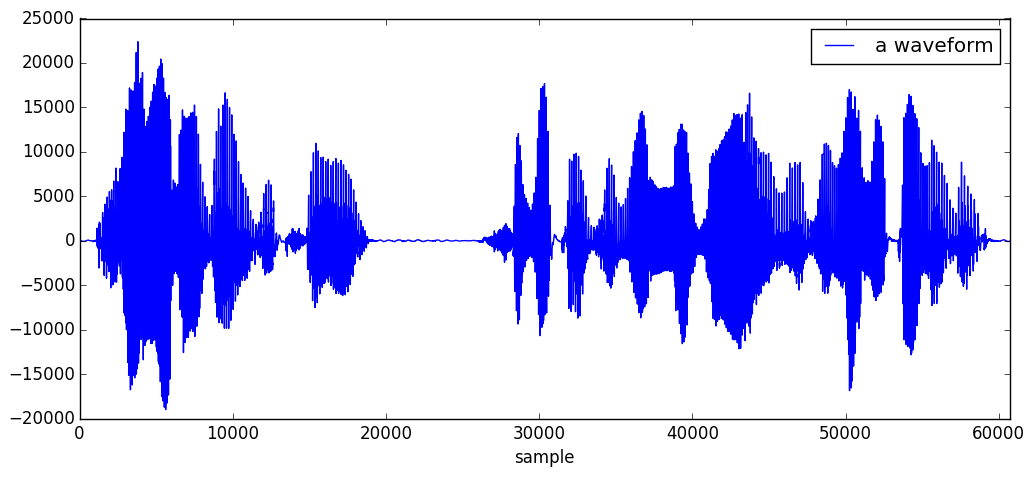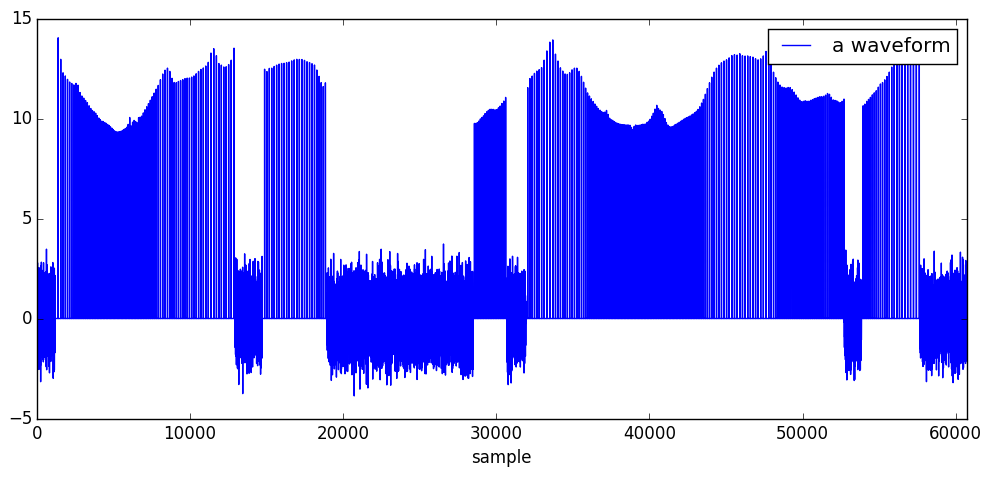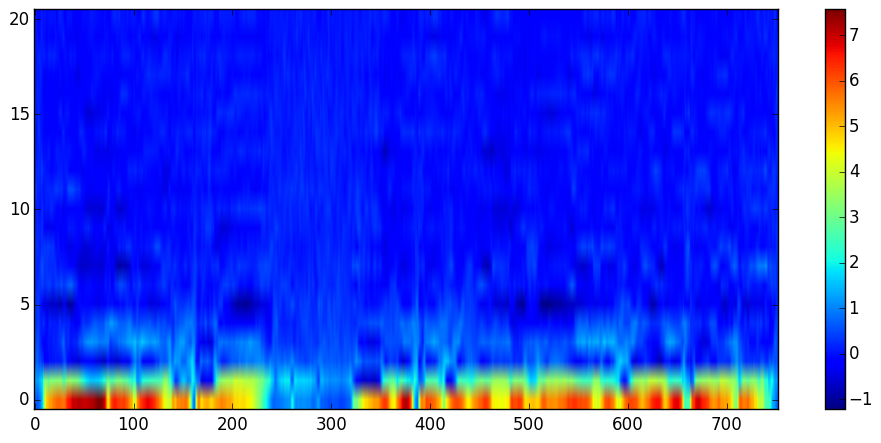# Introduction to SynthesisFilters.jl¶

This notebook demonstrates how SyntheisFilters.jl works. Below we provide synthesized audio examples (Japanese) so that you are able to compare synthesis filters on your browser. Please read on.

In this notebook, the following synthesis fileters are demonstrated.

• LMADF: Log magnitude approximation digital filter for synthesis from cepstrum
• MLSADF: Mel-log spectrum approximation digital filter for synthesis from mel-cepstrum
• MGLSADF: Mel generalized-log spectrum approximation digital filter for synthesis from mel-generalized cepstrum
• AllPoleDF: All-pole digital filter for synthesis from LPC
• AllPoleLatticeDF: All-pole lattice digital filter for synthesis from PARCOR
• LSPDF: LSP digital filter for synthesis from LSP
• MelGeneralizedCepstrums.jl: provides spectral parameter estimation based on mel-generalized cepstrum analysis.
• SPTK.jl: a thin wrapepr for SPTK
• WORLD.jl: a high-quality speech analysis, modification and synthesis system
In :
using PyCall
matplotlib = pyimport("matplotlib")
PyDict(matplotlib["rcParams"])["figure.figsize"] = (12, 5)
using PyPlot

WARNING: using PyPlot.matplotlib in module Main conflicts with an existing identifier.

In :
# https://gist.github.com/jfsantos/a39ed69a7894876f1e04#file-audiodisplay-jl
# Thanks, @jfsantos
include("AudioDisplay.jl")

Out:
inline_audioplayer (generic function with 2 methods)
In :
using WAV
using DSP
using MelGeneralizedCepstrums # to esimate spectral envelope parameters
using SynthesisFilters

In :
# plotting utilities
function wavplot(x; label="a waveform", x_label="sample")
plot(1:endof(x), x, "b", label=label)
xlim(1, endof(x))
xlabel(x_label)
legend()
end

function wavcompare(x, y; label="synthesized waveform", x_label="sample")
plot(1:endof(y), y, "r-+", label=label)
plot(1:endof(x), x, label="original speech signal")
xlim(1, endof(x))
xlabel(x_label)
legend()
end

Out:
wavcompare (generic function with 1 method)

## Data¶

In this notebook, we use the follwoing audio data to analyze and re-synthesize. Let's see and listen the example.

In :
x, fs = wavread(joinpath(dirname(@__FILE__), "data", "test16k.wav"), format="native")
x = convert(Vector{Float64}, vec(x))
fs = convert(Int, fs)
wavplot(x)
inline_audioplayer(map(Int16, x), fs)## Speech parameter extraction¶

To syntheisze a wavefrom, basically you need two speech parameters:

• excitation signal
• spectral parameters (e.g. Mel-cepstrum)

MelGenralizedCepstrums.jl supports extracting lots of spectral parameters.

### Excitation signal¶

In this notebook, we use a pre-extracted excitation signal, for test16k.wav in the example directory.

In :
# Note about excitation
# fs: 16000
# frame period: 5.0 ms
# F0 analysis: esimated by WORLD.dio and WORLD.stonemask
# Excitation genereration: perioic pulse for voiced segments and gaussian random
# values for un-voiced segments
wavplot(base_excitation)
inline_audioplayer(base_excitation ./ maximum(base_excitation), fs)## Split audio signal into overlapping time frames and apply windowing¶

This ia a basic step before mel-genrealized cesptrum analysiis. Note that windowing is essential for mel-generalized cepstrum analysis.

In :
framelen = 512
hopsize = 80 # 5.0 ms for fs 16000
noverlap = framelen - hopsize

# Note that mgcep analysis basically assumes power-normalized window so that Σₙ w(n)² = 1
win = DSP.blackman(framelen) ./ sqrt(sumabs2(DSP.blackman(framelen)))
@assert isapprox(sumabs2(win), 1.0)

# create windowed signal matrix that each column represents a windowed time slice
as = arraysplit(x, framelen, noverlap)
xw = Array(Float64, framelen, length(as))
for t=1:length(as)
xw[:,t] = as[t]
end

# col-wise windowing
xw .*= win;
@show size(xw)

size(xw) = (512,753)
Out:
(512,753)

## Spectral parameter estimation¶

You can extact lots of spectral parameters using MelGenrealizedCepstrums.jl. In the follwoing example, we extract mel-cepstrum from the windowed signal and then show the spectral envelope estimte.

In :
c = estimate(MelCepstrum(20, mcepalpha(fs)), xw)
imshow(c, origin="lower", aspect="auto")
colorbar()Out:
PyObject <matplotlib.colorbar.Colorbar instance at 0x7fcc0f712998>

In :
# Let's see spectral envelope estimate
imshow(real(mgc2sp(c, framelen)), origin="lower", aspect="auto")
colorbar()